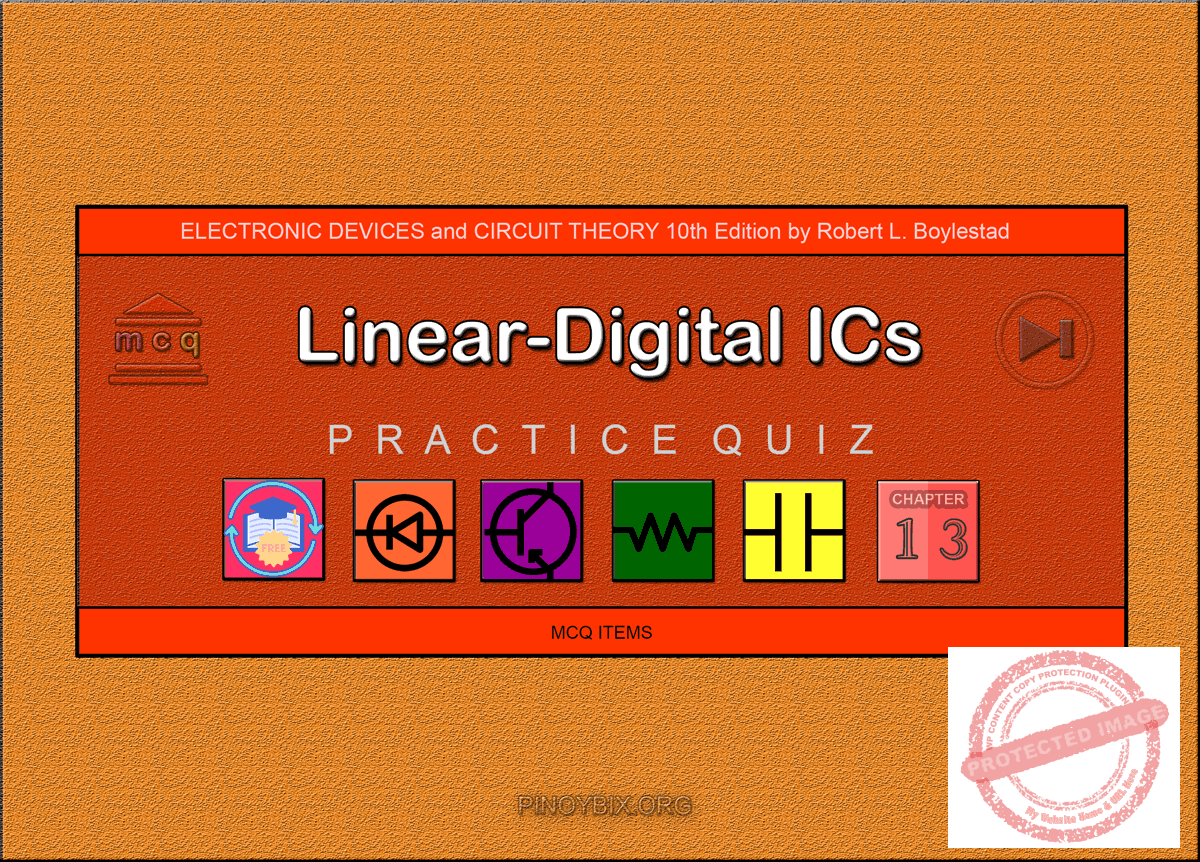(Last Updated On: February 4, 2020)Below are the answers key for the Multiple Choice Questions (Quiz) in Linear-Digital ICs from the book Electronic Devices and Circuit Theory 10th Edition by Robert L. Boylestad.

1. C. Passive filter

2. D. All of the above

3. D. All of the above

4. A. 4

5. A. comparator

6. A. comparator

7. A. comparator

8. C. Changing a digital signal to an analog signal

9. C. 0 V or Vref

10. A. The analog output voltage proportional to the digital input voltage

11. B. Vref /256

12. C. Both positive and negative

13. A. Connecting the analog voltage to the integrator for a fixed time

14. C. At the end of the charging of the capacitor

15. D. All of the above

16. B. During the discharging of the capacitor of the integrator

18. D. 1.024 ms

19. B. 976

20. A. Comparator

23. D. 128 Hz

24. A. True

25. B. Astable multivibrator

26. A. Monostable multivibrator

27. C. Both square- and triangular-wave

28. D. All of the above

29. B. 53.33 kHz

30. C. 116.39 kHz

31. A. True

32. D. All of the above

33. C. 2

34. A. 90.91 kHz

35. C. Both 1070 Hz and 1270 Hz

#### FILL-IN-THE-BLANKS

1. A. linear, digital

2. C. either the plus or minus, the other

3. D. All of the above

4. C. either a dual power supply of 15 V or a single +5 V supply

5. B. any positive, low

6. D. within the high and the low reference voltages

7. C. either above the higher reference voltage or below the lower reference voltage

8. A. more, greater

9. B. comparator

10. D. 19.5 mV

11. A. 2VCC / 3, VCC / 3

12. C. RA and RB, RB

13. D. 1/3 VCC, 2/3 VCC

14. C. microseconds, many seconds

15. B. varying

16. C. both an external resistor and an external capacitor

17. D. All of the above

18. A. the same as

19. B. remove the sum frequency component

20. B. an external resistor

21. B. 14 V

22. A. high

23. D. 5 V, 0 V

24. B. –12 V, 12 V

25. D. All of the above